# Volume Of A Hemisphere

Here we will learn about the volume of a hemisphere.

There are also volume of a hemisphere worksheets based on Edexcel, AQA and OCR exam questions, along with further guidance on where to go next if you’re still stuck.

## What is the volume of a hemisphere?

The volume of a hemisphere is the amount of space inside a hemisphere.

To calculate the volume of a hemisphere, we need to know what a hemisphere is. A hemisphere is half of a sphere. It has a radius, r.

To calculate the volume of a whole sphere, where r is the radius of the sphere, we use the formula

\text{Volume of a sphere}=\frac{4}{3} \pi r^3.

Since a hemisphere is half of a sphere, to find the volume of a hemisphere we halve the volume of a sphere.

Here is the volume of a hemisphere formula, with radius r

\text{Volume of a hemisphere}=\frac{4}{3} \pi r^3\div 2.

Or, alternatively,

\begin{aligned} \text { Volume of a hemisphere } &=\frac{1}{2} \times \frac{4}{3} \pi r^{3} \\\\ &=\frac{2}{3} \pi r^{3}. \end{aligned}

### What is the volume of a hemisphere?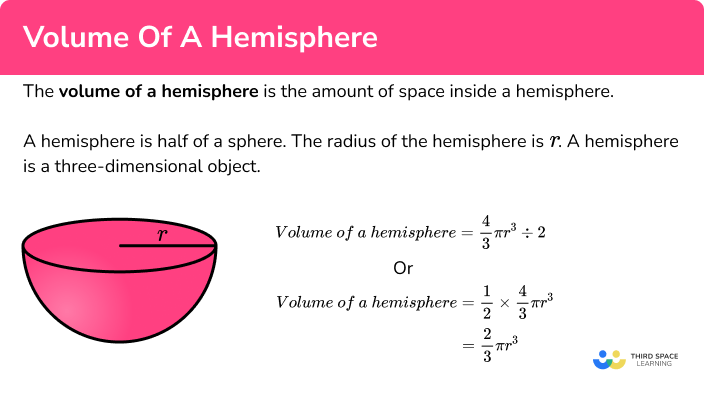## How to calculate the volume of a hemisphere

In order to calculate the volume of a hemisphere:

1. Write down the formula for the sphere.
2. Adapt the formula for the question.
3. Substitute in the value.

### Explain how to calculate the volume of a hemisphere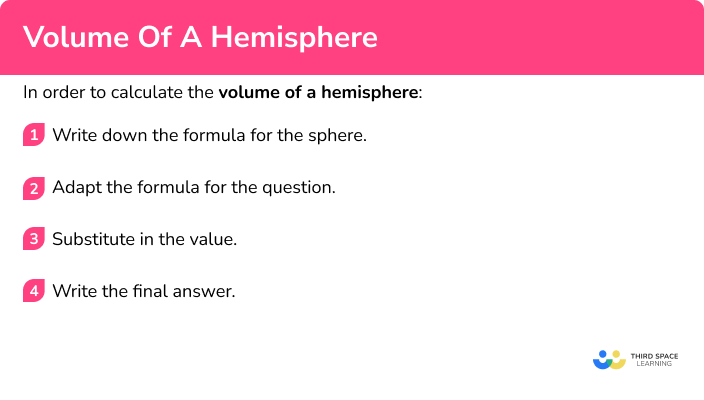## Related lessons on hemisphere shape

Volume of a hemisphere is part of our series of lessons to support revision on hemisphere shape. You may find it helpful to start with the main hemisphere shape lesson for a summary of what to expect, or use the step by step guides below for further detail on individual topics. Other lessons in this series include:

## Volume of a hemisphere examples

### Example 1: radius is given

Calculate the volume of a hemisphere with radius 4.5 \ cm. Give your answer to 1 decimal place.

1. Write down the formula for the sphere.

The formula for the volume of a sphere is

V=\frac{4}{3} \pi r^3.

2Adapt the formula for the question.

The formula for finding the volume of a hemisphere is

V=\frac{4}{3} \pi r^3\div 2.

3Substitute in the value.

V=\frac{4}{3} \pi (4.5)^3\div 2

This rounds to give the volume 190.9 \ cm^3 to 1 decimal place.

### Example 2: diameter of a hemisphere is given

Calculate the volume of a hemisphere with diameter 12.3 \ cm. Give your answer to 1 decimal place.

The formula for the volume of a sphere is

V=\frac{4}{3} \pi r^3.

The formula for finding the volume of a hemisphere is

V=\frac{4}{3} \pi r^3\div 2.

We have been given the diameter. We need to halve 12.3 to get the radius.

r=12.3\div 2=6.15

V=\frac{4}{3} \pi (6.15)^3\div 2

This rounds to give the volume 487.2 \ cm^3 to 1 decimal place.

Calculate the volume of a hemisphere with radius 6 \ cm. Leave your answer in terms of \pi.

The formula for the volume of a sphere is

V=\frac{4}{3} \pi r^3.

The formula for finding the volume of a hemisphere is

V=\frac{4}{3} \pi r^3\div 2.

V=\frac{4}{3} \pi (6)^3\div 2=\frac{4}{3}\times \pi \times 216 \div 2

144\pi \ cm^3.

### Example 4: leaving answer in terms of pi, diameter of a hemisphere is given

Calculate the volume of a hemisphere with diameter 6 \ cm. Leave your answer in terms of \pi .

The formula for the volume of a sphere is

V=\frac{4}{3} \pi r^3.

The formula for finding the volume of a hemisphere is

V=\frac{4}{3} \pi r^3\div 2.

We have been given the diameter. We need to halve 6 to get the radius.

r=6\div 2=3

V=\frac{4}{3} \pi (3)^3\div 2=\frac{4}{3}\times \pi \times 27 \div 2

18\pi \ cm^3.

### Example 5: finding the radius, given the volume

The volume of a hemisphere is 500 \ cm^{3}. Calculate the radius. Give your answer to 3 significant figures.

The formula for the volume of the sphere is

V=\frac{4}{3} \pi r^3.

The formula for finding the volume of a hemisphere is

V=\frac{4}{3} \pi r^3\div 2.

We can substitute in the value of the volume.

500=\frac{4}{3} \pi r^3\div 2

Then we rearrange the equation to solve it and find the radius.

\begin{aligned} 500&= \frac{4}{3}\pi r^3 \div 2 \\\\ 1000&= \frac{4}{3}\pi r^3 \\\\ 750&=\pi r^3\\\\ \frac{750}{\pi}&=r^3\\\\ r&=\sqrt{\frac{750}{\pi}} \end{aligned}

This rounds to give the radius as 6.20 \ cm to 3 significant figures.

### Example 6: finding the diameter, given the volume

The volume of a hemisphere is 400 \ cm^{3}. Calculate the diameter. Give your answer to 3 significant figures.

The formula for the volume of a sphere is

V=\frac{4}{3} \pi r^3.

The formula for finding the volume of a hemisphere is

V=\frac{4}{3} \pi r^3\div 2.

We can substitute in the value of the volume.

400=\frac{4}{3} \pi r^3\div 2

Then we rearrange the equation to solve it and find the radius.

\begin{aligned} 400&= \frac{4}{3}\pi r^3 \div 2 \\\\ 800&= \frac{4}{3}\pi r^3 \\\\ 600&=\pi r^3\\\\ \frac{600}{\pi}&=r^3\\\\ r&=\sqrt{\frac{600}{\pi}} \end{aligned}

This gives the radius as 5.7588…, which needs to be doubled to find the diameter.

This rounds to give the diameter as 11.5 \ cm to 3 significant figures.

### Common misconceptions

• Using the correct formula

There are several formulas that can be used, so we need to match the correct formula to the correct context.

• Rounding

It is important to not round the answer until the end of the calculation. This will mean your final answer is accurate. It is useful to keep your answer in terms of until you round the answer at the very end of the question.

• Make sure you have the correct units

For area we use square units such as cm^{2}.

For volume we use cubic units such as cm^{3}.

• Using the radius or the diameter

It is a common error to mix up radius and diameter. Remember the radius is half of the diameter.

### Practice volume of a hemisphere questions

1. Find the volume of a hemisphere with radius 7.9 \ cm. Give your answer correct to 1 decimal place.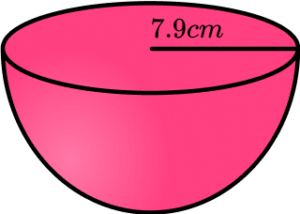1032.6 \ cm^32065.2 \ cm^32065.3 \ cm^31032.7 \ cm^3The volume of the hemisphere can be found by using the volume of a sphere and halving it.

\begin{aligned} V&=\frac{4}{3} \pi r^3\div 2\\\\ V&=\frac{4}{3} \pi (7.9)^3\div 2\\\\ V&=1032.618…\\\\ V&=1032.6 \ cm^3 \ \text{(to 1 dp)} \end{aligned}

2. Find the volume of a hemisphere with diameter 4.6 \ cm. Give your answer correct to 1 decimal place.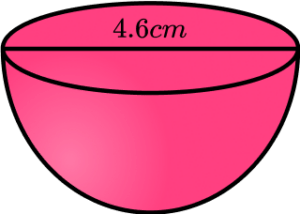25.4 \ cm^3203.8 \ cm^3203.9 \ cm^325.5 \ cm^3First we need to divide the diameter by 2 to find the radius. The radius is 2.3 \ cm. The volume of the hemisphere can be found by using the volume of a sphere and halving it.

\begin{aligned} V&=\frac{4}{3} \pi r^3\div 2\\\\ V&=\frac{4}{3} \pi (2.3)^3\div 2\\\\ V&=25.4825…\\\\ V&=25.5 \ cm^3 \ \text{(to 1 dp)} \end{aligned}

3. Find the volume of a hemisphere with radius 10 \ cm. Leave your answer in terms of \pi .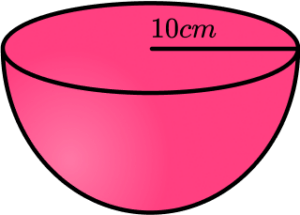\frac{2000}{3}\pi \ cm^31000\pi \ cm^3\frac{1000}{3}\pi \ cm^32000\pi \ cm^3The volume of the hemisphere can be found by using the volume of a sphere and halving it.

\begin{aligned} V&=\frac{4}{3} \pi r^3\div 2\\\\ V&=\frac{4}{3} \pi (10)^3\div 2\\\\ V&=\frac{2000}{3}\pi\\\\ V&=\frac{2000}{3}\pi \ cm^3 \end{aligned}

4. Find the volume of a hemisphere with diameter 4 \ cm. Leave your answer in terms of \pi .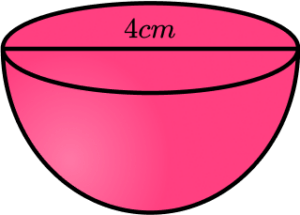\frac{256}{3}\pi \ cm^3\frac{16}{3}\pi \ cm^3\frac{32}{3}\pi \ cm^3\frac{128}{3}\pi \ cm^3First we need to divide the diameter by 2 to find the radius. The radius is 2 \ cm. The volume of the hemisphere can be found by using the volume of a sphere and halving it.

\begin{aligned} V&=\frac{4}{3} \pi r^3\div 2\\\\ V&=\frac{4}{3} \pi (2)^3\div 2\\\\ V&=\frac{16}{3}\pi\\\\ V&=\frac{16}{3}\pi \ cm^3 \end{aligned}

5. A hemisphere has a volume of 1500 \ cm^{3}. Calculate its radius. Give your answer correct to 3 significant figures.

17.8 \ cm17.9 \ cm8.94 \ cm8.95 \ cmFirst we adapt the formula for the volume of a sphere by dividing it by 2 to give the volume of a hemisphere.

V=\frac{4}{3} \pi r^3\div 2

Then we form an equation by substituting the volume and rearrange it to find the radius.

\begin{aligned} 1500&= \frac{4}{3}\pi r^3 \div 2 \\\\ 3000&= \frac{4}{3}\pi r^3 \\\\ 2250&=\pi r^3\\\\ \frac{2250}{\pi}&=r^3\\\\ r&=\sqrt{\frac{2250}{\pi}}\\\\ r&=8.94700…\\\\ r&=8.95 \ cm \ \text{(to 3 sf)} \end{aligned}

6. A hemisphere has a volume of 2300 \ cm^{3}. Calculate its diameter. Give your answer correct to 3 significant figures.

10.3 \ cm20.6 \ cm10.4 \ cm20.7 \ cmFirst we adapt the formula for the volume of a sphere by dividing it by 2, to give the volume of a hemisphere.

V=\frac{4}{3} \pi r^3\div 2

Then we form an equation by substituting the volume and rearrange it to find the radius. Finally we multiply the radius by 2 to find the diameter.

\begin{aligned} 2300&= \frac{4}{3}\pi r^3 \div 2 \\\\ 4600&= \frac{4}{3}\pi r^3 \\\\ 3450&=\pi r^3\\\\ \frac{3450}{\pi}&=r^3\\\\ r&=\sqrt{\frac{3450}{\pi}}\\\\ r&=10.317…\\\\ d&=20.6341… \\\\ d&=20.6 \ cm \ \text{(to 3 sf)} \end{aligned}

### Volume of hemisphere GCSE questions

1. Find the volume of a hemisphere with radius 24 \ cm.

(2 marks)

Substituting 24 \ cm into V=\frac{4}{3} \pi r^{3} \div 2 .

(1)

9216\pi \ cm^3

(1)

2. Susan has a sculpture in her garden. It is made of a cube and a hemisphere. The diameter of the hemisphere is 2 metres.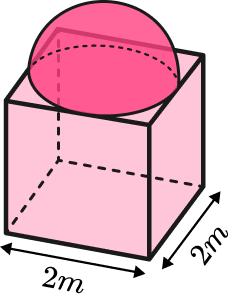Calculate the total volume of the sculpture. Give your answer to 1 decimal place.

(3 marks)

\frac{4}{3}\times \pi \times 1^3 \div 2=\frac{2}{3}\pi

(1)

\frac{2}{3}\pi +2^3

(1)

10.09439… = 10.1 \ m^3

(1)

3. A hemisphere has a volume of 270 \ cm^3.

Find the radius of the hemisphere.

(3 marks)

270=\frac{4}{3}\pi{r}^{3}\div 2

(1)

r=\sqrt{\frac{270\times 2 \times 3}{4\pi}}

(1)

4.00951… = 4.01 \ cm

(1)

## Learning checklist

You have now learned how to:

• Find the volume of a hemisphere
• Find the radius of a hemisphere
• Solve problems involving the volume of a hemisphere

## Still stuck?

Prepare your KS4 students for maths GCSEs success with Third Space Learning. Weekly online one to one GCSE maths revision lessons delivered by expert maths tutors.

Find out more about our GCSE maths tuition programme.